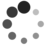# [c-faq] 2.14, 2.15-offsetof和struct成员异类访问

by PingboWen of TinyLab.org 2013/08/24

``````*(int *)((char *)structp + offsetf) = value;
``````

``````struct test {
char c;
int i;
};
``````

``````/* Offset of member MEMBER in a struct of type TYPE. */
#define offsetof(TYPE, MEMBER) __builtin_offsetof (TYPE, MEMBER)
``````

``````#define offsetof(type,m) ((size_t)&(((type *)0)->m))
``````

``````#define offsetof(type,m) ( (size_t) (((char *)&(((type *)0)->m)) - (char *)((type *)0)) )
``````

OK ，清楚了 offsetof 的实现后，咱再回过头来看看那个 struct 成员的异类访问：

``````struct test {
char c;
int i;
long l;
double t;
};
struct test st;
``````

``````st.t=7.89;
``````

``````*(double *)((char *)&st+offsetof(struct test, t))=7.89;
``````

``````#define container_of(ptr, type, member) ({ \
const typeof( ((type *)0)->member ) *__mptr = (ptr); \
(type *)( (char *)__mptr - offsetof(type,member) );})
``````

``````test.c:

#include <stdio.h>
//#define offsetof(type,m) ((size_t)&(((type *)0)->m))
#define offsetof(type,m) ( (size_t) (((char *)&(((type *)0)->m)) - (char *)((type *)0)) )

struct test {
char c;
int i;
long l;
double t;
};

int main(int argc,char *argv[])
{
struct test st;

printf("t pre: %f\n", st.t);

*(double *)((char *)&st+offsetof(struct test, t))=7.89;
printf("i after:%f\n", st.t);

return 0;
}
``````